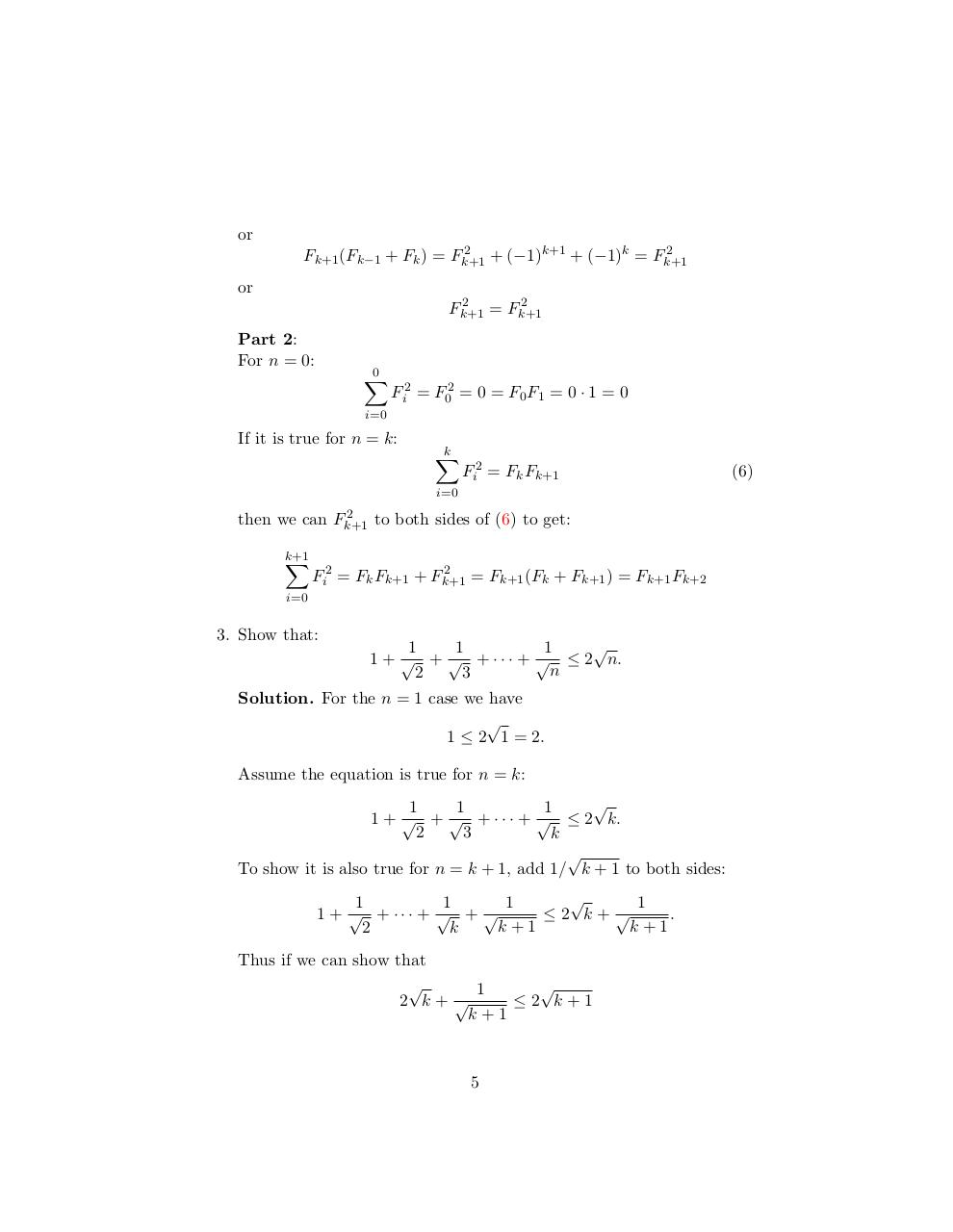# induction.pdfPage 1 2 3 4 5 6 7 8

#### Text preview

or
2
2
Fk+1 (Fk−1 + Fk ) = Fk+1
+ (−1)k+1 + (−1)k = Fk+1

or
2
2
Fk+1
= Fk+1

Part 2:
For n = 0:

0
X

Fi2 = F02 = 0 = F0 F1 = 0 · 1 = 0

i=0

If it is true for n = k:

k
X

Fi2 = Fk Fk+1

i=0
2
then we can Fk+1
to both sides of (6) to get:
k+1
X

2
= Fk+1 (Fk + Fk+1 ) = Fk+1 Fk+2
Fi2 = Fk Fk+1 + Fk+1

i=0

3. Show that:

1
1
1
1 + √ + √ + · · · + √ ≤ 2 n.
n
2
3

Solution. For the n = 1 case we have

1 ≤ 2 1 = 2.
Assume the equation is true for n = k:

1
1
1
1 + √ + √ + · · · + √ ≤ 2 k.
2
3
k

To show it is also true for n = k + 1, add 1/ k + 1 to both sides:

1
1
1
1
1 + √ + ··· + √ + √
≤2 k+ √
.
k+1
k+1
2
k
Thus if we can show that

1
2 k+ √
≤2 k+1
k+1

5

(6)